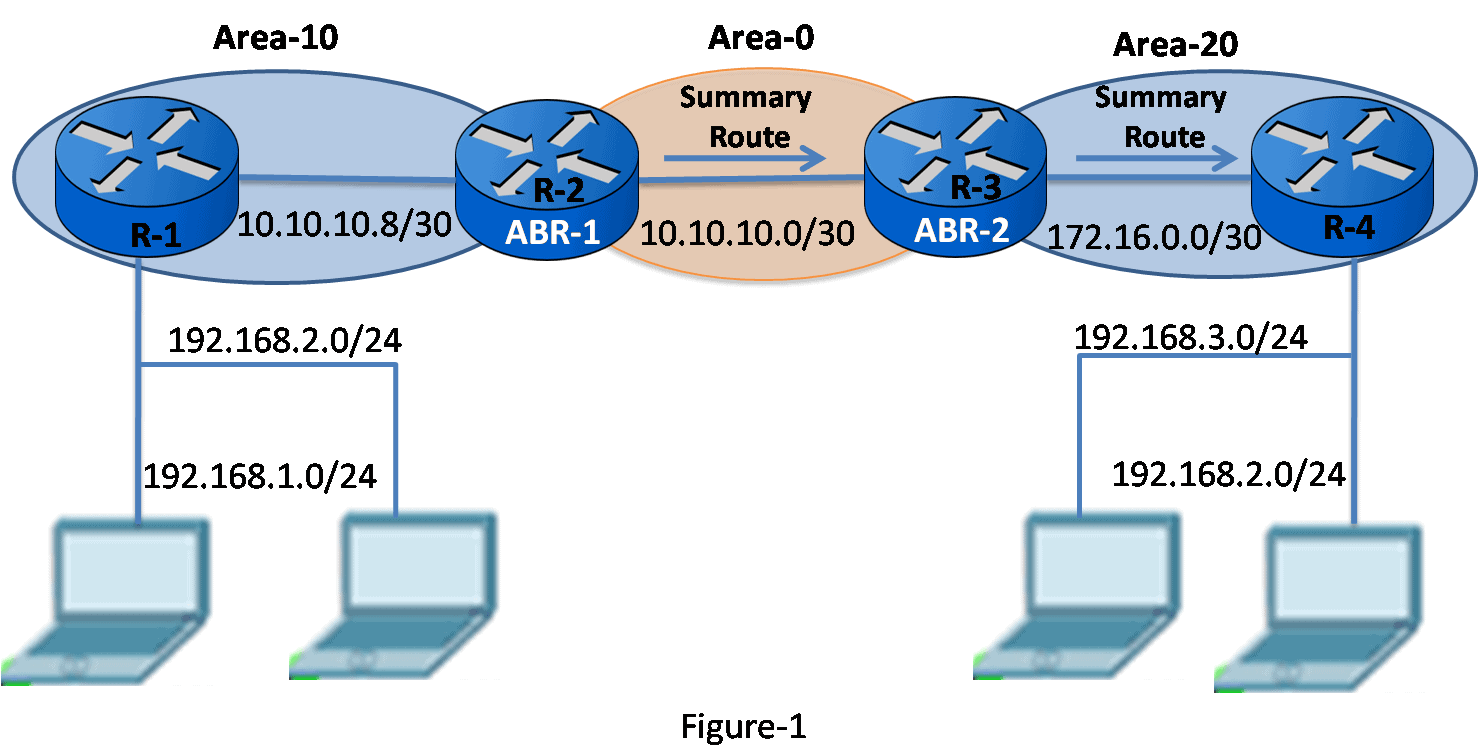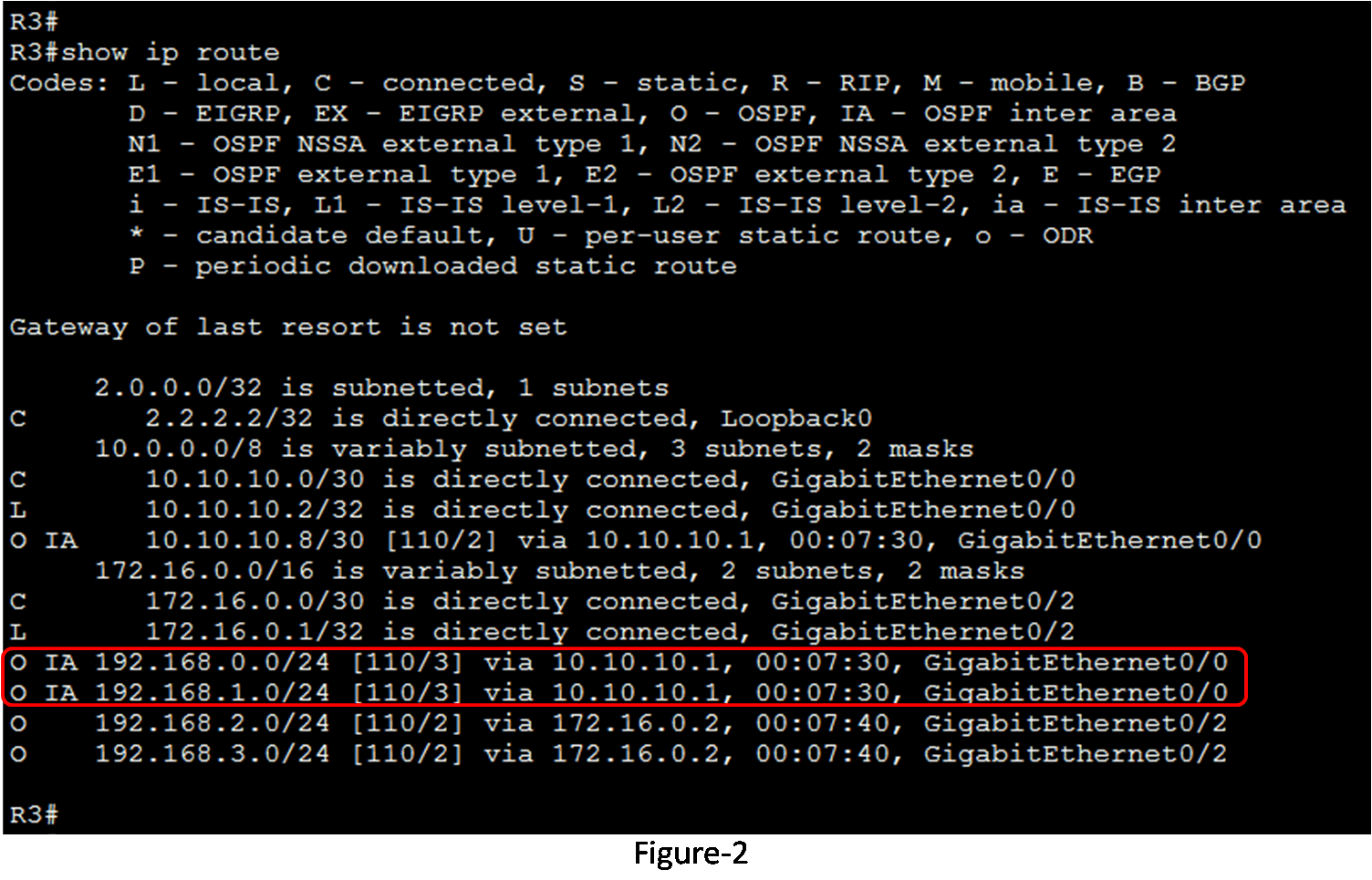# Interarea Route Summarization

Interarea route summarization in the OSPF must be manually configured on ABRs because OSPF does not support auto-summarization. We can perform internal routes summarization only on ABRs. When route summarization is configured on ABRs, a single type 3 LSA (summary LSA) describing the summary route are forwarded to the backbone area.

Multiple routes inside the area are summarized by the single LSA. A summarized route is generated if at least one subnet within the area comes down in the summary-address range. The summarized route metric is the same as the lowest cost of all subnets in the summary-address range. The ABR can only sum up routes that are within the areas connected to the ABR.

Figure 1 illustrates a multiarea OSPF topology. Figure 2 illustrates the routing tables of R3 before the route summarization is configured on R2.## Calculating the Summary Route

Summarizing different adjacent subnetworks into a single address and mask can calculate in three steps. The steps are the following:-

1. List all networks in binary format. For example, the two networks of area 10, 192.168.0.0/24 and 192.168.1.0/24 are listed in binary format in figure 3 (1).
2. Count the matching bits from left to right. The matching bits determine the mask for the summary route. As masked, the first 23 far left bits match. This results in the prefix/23 or subnet mask 255.255.254.0. Figure 3(2) illustrates the counting of matching bits.
3. Copy the matching bits from left to right and then add zero bits to remaining positions to determine the summarized network address as shown in figure 3(3). In this example, the matching bits with zeros at the result is a network address of 192.168.0.0/23. This summary-address summarizes two networks: 192.168.0.024, and 192.168.1.0/24. In the example the two networks matched out of three networks.## Configuring Interarea Route Summarization

We can manually configure the route summarization on ABRs. The R2 is ABR for area 10 and area 0. In the example, R1 is configured to summarize the internal area 10 routes.

To configure route summarization manually on ABR, use the area <area-id> range <address> <mask> command on router configuration mode. This command instructs the ABR to summarize routes for a specific area before injecting them into a different area, via the backbone as type 3 summary LSAs. In our example the configuration on R2 is:

• R2(config)#router ospf 10
• R2(config-router)# area 10 range 192.168.0.0 255.255.254.0
• R2(config-router)# do wr

In OSPFv3, the command is the same except for the IPv6 network address. The command syntax for OSPFv3 is area area-id range prefix/prefix-length.

Figure 4 displays the updated R3 routing table. You can examine that there is only one interarea route entry now available in the routing table for both routes. Though this example only reduced the routing table by one entry, summarization could be implemented, to sum up a lot of networks to reduce the size of routing tables extremely.(Visited 39 times, 39 visits today)